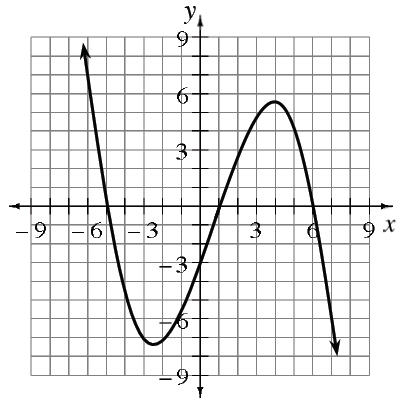### Home > APCALC > Chapter 4 > Lesson 4.3.2 > Problem4-123

4-123.

Refer to the graph at right of $y = f^\prime\left(x\right)$, the derivative of some function $f$.

1. Where is $f$ increasing? How can you tell?

Notice that this is the graph of $f^\prime\left(x\right)$, the slopes of $f\left(x\right)$, and $f\left(x\right)$ will increase where its slopes are positive.

2. Approximate the interval over which $f$ is concave up. Justify your conclusion with the graph.

Eyeball to see where you believe the point of inflections are in order to approximate the intervals.  Note: Concave up is a U shape.

3. Is $f^{\prime\prime}\left(0\right)$ positive or negative? Explain how you know.

$f^{\prime\prime}\left(x\right)$ represents the slopes of the given graph, $f ^\prime\left(x\right)$. So look at the graph. Does it have a positive or a negative slope at $x = 0$?